Mech. Sci., 13, 427–436, 2022
https://doi.org/10.5194/ms-13-427-2022

Mech. Sci., 13, 427–436, 2022
https://doi.org/10.5194/ms-13-427-2022
Research article | 04 May 2022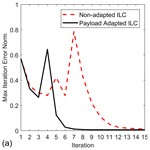Kaloyan Yovchev and Lyubomira Miteva Kaloyan Yovchev and Lyubomira Miteva
• Faculty of Mathematics and Informatics, Sofia University, Sofia, Bulgaria

Correspondence: Kaloyan Yovchev (k.yovchev@fmi.uni-sofia.bg)

Abstract

Share
1 Introduction

Industrial robotic arm manipulators are machines that must track trajectories with high accuracy. This trajectory tracking process depends on the correct estimation of the parameters of the dynamics of both the robot itself and its payload. As a payload both the end effector and the object with which the robot interacts may be considered. Even if the dynamics of the robot is estimated precisely, the control system will have to compensate for the error introduced by the unknown dynamics or the variation of the dynamics of the payload. These uncertainties of variations will introduce trajectory tracking errors.

ILC is a non-dual kind of adaptive control that was first proposed by Uchiyama (1978) and further developed by Arimoto et al. (1984a, b) for systems that function in a repeatable pattern. The ILC procedure will begin by making an educated guess about the required output control signals, which will be generated using the approximated mathematical parameters of the model. The ILC will thereafter require multiple iterations. The approach evaluates the tracking error after each iteration and adjusts the output control signals using a particular learning operator. When a pre-set acceptable accuracy threshold is reached, the iterative control procedure will be terminated. Consequently, a biologically inspired iterative self-learning process is created.

The ILC must generate a convergent process before it can be applied to a robot system. In today's literature, the convergence of the ILC approach has been thoroughly researched. Heizinger et al. (1989) proved the convergence of the ILC when non-linear systems (such as robotic manipulators) are considered. Longman and Huang (2002) observed an issue with the formation of a transient error during the first few iterations. This issue necessitated more investigation due to the mechanical features of the robotic manipulators which constrain their state space. This issue prevents the ILC from being directly applicable to constrained state space robotic manipulators. The constrained output ILC (COILC) method, described in Yovchev et al. (2020a), can be applied for control of robotic manipulators without imposing any extra constraints on their workspace, as well as to solve the problem of transient growth error. Robustness and convergence of the COILC are proven when an estimation of the inertia matrix is used as a learning operator.

The focus of today's ILC investigation is on improving the overall performance of the ILC and for reducing the required number of ILC executions (iterations) for generating the control signals for precise trajectory tracking when payload variations are considered. The performance of the ILC methods can be improved by a range of machine learning methods (Wei-Liang et al., 2017; Zhang et al., 2019). The work of Nemec et al. (2017) provides an adaptive ILC method to achieve smooth and safe manipulation of fragile items, with the adaptation supervised by reinforcement learning. Neural networks (Patan et al., 2017; Xu and Xu, 2018) or fuzzy neural network methods (Wang et al., 2008; Wang et al., 2014) are used within ILC to reduce the uncertainty of the model used for the design of the controller. The basis-motion torque composition approach (Sekimoto et al., 2009) has been proposed as a solution for the main disadvantage of the ILC, that the ILC requires a new learning process for achieving a different motion (Tanimoto et al., 2017).

This paper is organized as follows. Section 2 formulates the problem of applying ILC to trajectory tracking of a robotic manipulator with a payload and proposes an approach for ILC adaption for a specific payload. Section 3 validates the proposed algorithm through a numerical experiment and presents a computer simulation. Section 4 summarizes the results and proposes future research directions.

Figure 1 shows the standard ILC scheme. P denotes the robot arm, L represents the learning controller, M represents the control's system memory, $l=\mathrm{1},\mathrm{\dots },N$ denotes the current iteration number, N represents the total number of iterations, ul represents control law's the feed-forward term for iteration l, ql denotes the output trajectory for iteration l, and qd represents the desired output trajectory. After each iteration, the feed-forward term ul+1 is computed offline and is used to reduce the trajectory tracking error for iteration l+1.

The synthesis of the learning update law of the conventional ILC requires the definition of feed-forward and feedback controllers. The update control law iteratively improves the feed-forward control term by the following rule:

$\begin{array}{}\text{(1)}& {\mathbit{u}}_{l+\mathrm{1}}={\mathbit{u}}_{l}+L\left({\mathbit{q}}_{l}\right)\left({\stackrel{\mathrm{¨}}{\mathbit{q}}}_{\mathrm{d}}-{\stackrel{\mathrm{¨}}{\mathbit{q}}}_{l}\right),\end{array}$

where L(ql(t)), $l=\mathrm{0},\mathrm{1},\mathrm{\dots },N$ is a learning operator, u0(t)≡0 is the initial feed-forward control input, ${\stackrel{\mathrm{¨}}{\mathbit{q}}}_{\mathrm{d}}$ are the desired joint torques, ${\stackrel{\mathrm{¨}}{\mathbit{q}}}_{l}$ is the output joint torque during iteration l, and $t\in \left[\mathrm{0},T\right]$ denotes time, where [0, T] is the trajectory tracking time interval.

The COILC procedure described by Yovchev et al. (2020a) can be used for the control of any type of robotic manipulator with k joints and state space constraints (defined as ${Q}_{j}^{min}\le {q}_{j}\le {Q}_{j}^{max},\mathrm{1}\le j\le k\right)$, which is supposed to minimize the tracking error of any attainable desired trajectory qd:

• a.

Start with initial iteration number l=0, and proceed with the iterative procedure.

• b.

Starting from the initial position ql(0), the system tracks the desired trajectory under the control ul(t) until the first moment Sl for which there exists $j:\mathrm{1}\le j\le k$, and either ${q}_{j}^{l}\left({S}_{l}\right)={Q}_{j}^{min}$ or ${q}_{j}^{l}\left({S}_{l}\right)={Q}_{j}^{max}$ or the end position ql(T) is reached. When t=Sl, ${S}_{l}\in \left(\mathrm{0},\phantom{\rule{0.125em}{0ex}}T\right]$, the tracking process stops.

• c.

After the current tracking performance has finished, the learning controller updates the feed-forward control term according to the following learning update law:

• d.

Calculate the current maximum trajectory tracking error, defined as |δql(t)|. If the error is less than or equal to a predefined acceptable accuracy, then exit from the learning procedure, or else set $l=l+\mathrm{1}$, and go to step (b).

Let $\stackrel{\mathrm{^}}{\mathbf{A}}$ be the approximation of the inertia matrix A. Then the ILC is robust and convergent if the learning operator from Eq. (1) is chosen as $\mathbf{L}\left(\mathbit{q}\right)\equiv \stackrel{\mathrm{^}}{\mathbf{A}}\left(\mathbit{q}\right)$ (Delchev, 2012). The approximated inertia matrix evaluates how each joint's parameters influence robot motion. The convergence rate will be improved by a better estimation $\stackrel{\mathrm{^}}{\mathbf{A}}$ of A.

As stated in the research by Yovchev and Miteva (2021), the performance of the ILC method depends on the selection of the estimation. The research proposed an additional parameter of the COILC – the scalar learning gain s by which the matrix $\stackrel{\mathrm{^}}{\mathbf{A}}$ will be multiplied and the learning operator will become $\mathbf{L}\left(\mathbit{q}\right)\equiv s\stackrel{\mathrm{^}}{\mathbf{A}}\left(\mathbit{q}\right)$. This will alter the ${\mathbit{u}}_{l}^{\ast }\left(t,{S}_{l}\right)$ from the COILC update law (Eq. 2) as follows:

This scalar is used to adapt the ILC process to a specific set of trajectories. The goal of the current research is to propose a new approach for adaptation of the ILC method for the specific type of executed task. The robot's dynamics is dependent of the end effector of the robot. When the end effector is changed, the estimation of the inertia matrix must be recalculated.

This research will consider the well-known SEIKO Instruments SCARA-type robot TT-3000. The kinematics of this robot is shown in Fig. 2. The joint lengths are l1= 250 mm and l2= 220 mm. The dynamical equations of motions and the parameters of the dynamics of this robot are described in research by Shinji and Mita (1990).

The standard formulation of the dynamics of this robot is formulated by Shinji and Mita (1990) as follows:

$\begin{array}{}\text{(4)}& \begin{array}{rl}{a}_{\mathrm{11}}\left({q}_{\mathrm{2}}\right){\stackrel{\mathrm{¨}}{q}}_{\mathrm{1}}& +{a}_{\mathrm{12}}\left({q}_{\mathrm{2}}\right){\stackrel{\mathrm{¨}}{q}}_{\mathrm{2}}-\mathrm{2}{m}_{\mathrm{2}}{l}_{\mathrm{1}}{s}_{\mathrm{2}}\mathrm{sin}\left({q}_{\mathrm{2}}\right){\stackrel{\mathrm{˙}}{q}}_{\mathrm{1}}{\stackrel{\mathrm{˙}}{q}}_{\mathrm{2}}\\ & -{m}_{\mathrm{2}}{l}_{\mathrm{1}}{s}_{\mathrm{2}}\mathrm{sin}\left({q}_{\mathrm{2}}\right){\stackrel{\mathrm{˙}}{q}}_{\mathrm{2}}^{\mathrm{2}}+{D}_{\mathrm{1}}{\stackrel{\mathrm{˙}}{q}}_{\mathrm{1}}+{f}_{\mathrm{1}}={u}_{\mathrm{1}}\\ {a}_{\mathrm{12}}\left({q}_{\mathrm{2}}\right){\stackrel{\mathrm{¨}}{q}}_{\mathrm{1}}& +{a}_{\mathrm{22}}{\stackrel{\mathrm{¨}}{q}}_{\mathrm{2}}+{m}_{\mathrm{2}}{l}_{\mathrm{1}}{s}_{\mathrm{2}}\mathrm{sin}\left({q}_{\mathrm{2}}\right){\stackrel{\mathrm{˙}}{q}}_{\mathrm{1}}^{\mathrm{2}}\\ & +{D}_{\mathrm{2}}{\stackrel{\mathrm{˙}}{q}}_{\mathrm{2}}+{f}_{\mathrm{2}}={u}_{\mathrm{2}}.\end{array}\end{array}$

The inertia matrix A(q)=[aij(q2)], $\mathrm{1}\le ij\le \mathrm{2}$, where

$\begin{array}{}\text{(5)}& \begin{array}{rl}{a}_{\mathrm{11}}\left({q}_{\mathrm{2}}\right)& ={I}_{\mathrm{1}}+{m}_{\mathrm{1}}{s}_{\mathrm{1}}^{\mathrm{2}}+{I}_{\mathrm{2}}+{m}_{\mathrm{2}}\left({I}_{\mathrm{1}}^{\mathrm{2}}+{s}_{\mathrm{1}}^{\mathrm{2}}\right)\\ & +\mathrm{2}{m}_{\mathrm{2}}{l}_{\mathrm{1}}{s}_{\mathrm{2}}\mathrm{cos}\left({q}_{\mathrm{2}}\right)\\ {a}_{\mathrm{12}}\left({q}_{\mathrm{2}}\right)& ={I}_{\mathrm{2}}+{m}_{\mathrm{2}}{s}_{\mathrm{2}}^{\mathrm{2}}+{m}_{\mathrm{2}}{l}_{\mathrm{1}}{s}_{\mathrm{2}}\mathrm{cos}\left({q}_{\mathrm{2}}\right)\\ {a}_{\mathrm{21}}\left({q}_{\mathrm{2}}\right)& ={a}_{\mathrm{12}}\left({q}_{\mathrm{2}}\right)\\ {a}_{\mathrm{22}}\left({q}_{\mathrm{2}}\right)& ={a}_{\mathrm{22}}={I}_{\mathrm{2}}+{m}_{\mathrm{2}}{s}_{\mathrm{2}}^{\mathrm{2}},\end{array}\end{array}$

where for $i=\mathrm{1},\mathrm{2}$, Di is the viscous damping, fi is Coulomb friction, li is the link length, si is the position of the centre of mass, mi is the total mass of the link, Ii is the inertia of the link about its centre of mass, and ui is the driving torque of the link.

When we consider the attached payload, then the inertia matrix $\stackrel{\mathrm{‾}}{\mathbf{A}}\left(\mathbit{q}\right)=\left[{\stackrel{\mathrm{‾}}{a}}_{ij}\left({q}_{\mathrm{2}}\right)\right]$, $\mathrm{1}\le ij\le \mathrm{2}$ is as follows:

$\begin{array}{}\text{(6)}& \begin{array}{rl}& {\stackrel{\mathrm{‾}}{a}}_{\mathrm{11}}\left({q}_{\mathrm{2}}\right)={a}_{\mathrm{11}}\left({q}_{\mathrm{2}}\right)+{I}_{\mathrm{m}}+m\left({l}_{\mathrm{1}}^{\mathrm{2}}+{l}_{\mathrm{2}}^{\mathrm{2}}+\mathrm{2}{l}_{\mathrm{1}}{l}_{\mathrm{2}}\mathrm{cos}\left({q}_{\mathrm{2}}\right)\right)\\ & {\stackrel{\mathrm{‾}}{a}}_{\mathrm{12}}\left({q}_{\mathrm{2}}\right)={a}_{\mathrm{12}}\left({q}_{\mathrm{2}}\right)+{I}_{\mathrm{m}}+m\left({l}_{\mathrm{2}}^{\mathrm{2}}+{l}_{\mathrm{1}}{l}_{\mathrm{2}}\mathrm{cos}\left({q}_{\mathrm{2}}\right)\right)\\ & {\stackrel{\mathrm{‾}}{a}}_{\mathrm{21}}\left({q}_{\mathrm{2}}\right)={\stackrel{\mathrm{‾}}{a}}_{\mathrm{12}}\left({q}_{\mathrm{2}}\right)\\ & {\stackrel{\mathrm{‾}}{a}}_{\mathrm{22}}\left({q}_{\mathrm{2}}\right)={\stackrel{\mathrm{‾}}{a}}_{\mathrm{22}}={a}_{\mathrm{22}}+{I}_{\mathrm{m}}+m{l}_{\mathrm{2}}^{\mathrm{2}},\end{array}\end{array}$

where the payload is attached to the second link and has the following characteristics: mass m and inertia about its centre of mass Im.

The payload (the attached end effector) is supposed to change the dynamics characteristics of the robotic manipulator, as can be seen from Eqs. (5) and (6). This will lead to a different performance of the ILC process when different payloads are used. Also, for optimal ILC the inertia characteristics of the payload must be known. The goal of this research is to propose a new approach with an added ILC adaptation phase when a new payload is attached. The estimation $\stackrel{\mathrm{^}}{\mathbf{A}}\left(\mathbit{q}\right)=\left[{\stackrel{\mathrm{^}}{a}}_{ij}\left({q}_{\mathrm{2}}\right)\right]$, $\mathrm{1}\le ij\le \mathrm{2}$ of the inertia matrix A(q) of the robot without payload will be modified by execution of a predefined training trajectory when a new payload is attached.

The estimated inertia matrix $\stackrel{\mathrm{^}}{\mathbf{A}}\left(\mathbit{q}\right)$ considers how the characteristics of each joint affect the robot's motion. Correct estimation of this matrix will lead to a robust and convergent ILC process. The learning operator cannot be randomly chosen due to a slow or non-convergent ILC process. For the purposes of this research, we will assume that the inertia matrix $\stackrel{\mathrm{^}}{\mathbf{A}}$ is estimated correctly for a robotic manipulator with no payload. We will introduce two additional parameters of the COILC – the scalar learning multiplier gain s and fine-tuning gain p by which the matrix $\stackrel{\mathrm{^}}{\mathbf{A}}$ will be modified to be used as a learning operator: $\mathbf{L}\left(\mathbit{q}\right)\equiv s\stackrel{\mathrm{^}}{\mathbf{A}}\left(\mathbit{q}\right)+p$. This will alter the ${\mathbit{u}}_{l}^{\ast }\left(t,{S}_{l}\right)$ from the COILC update law (Eq. 2) as follows:

When a new payload is attached, the robot will execute several consequent ILC processes with a predefined reference trajectory. These consequent ILC executions will evaluate multiple pairs of parameters (s, p).

The COILC constrains the output trajectory at each iteration l, so that the state space constraints cannot be violated during the ILC procedure, and the adaptation step can be safely executed.

The performance of the proposed approach is evaluated through numerical experiments and computer simulation in the following section.

3 Numerical experiments and simulation results

For evaluation on the viability and applicability of the proposed payload-adaptive ILC, we conduct numerical experiments and a computer simulation with a specialized software. For the numerical experiments, we will use GNU Octave software to solve the ordinary differential equations of the dynamics (Eaton et al., 2019). For the computer simulations, we will use the Cyberbotics Ltd. Webots™ robotic simulator (Michel, 2004) and its simulation of the Universal Robots' UR5e robotic manipulator. The computer simulation will be used for better explanation of the advantages of the payload adaption of the ILC learning operator.

For the numerical experiments of the adaptive COILC algorithm, a computer simulation is used, as described in Sect. 2. The robot will have the following joint constraints ${Q}_{i}^{min}\le {q}_{i}\le {Q}_{i}^{max}$, where $\mathrm{1}\le i\le \mathrm{2}$ as described in Table 1.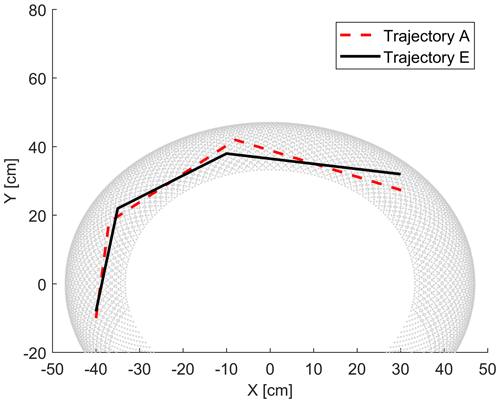Figure 4The grey dots represent the workspace of the robot. The dashed red and the solid black lines represent two similar desired trajectories A and E in the workspace.

The inertia matrix A from Eq. (5) of the simulated robot with no payload will be as follows:

$\begin{array}{}\text{(8)}& \begin{array}{rl}& {a}_{\mathrm{11}}\left({q}_{\mathrm{2}}\right)=\mathrm{6596.2}+\mathrm{3011.2}\mathrm{cos}\left({q}_{\mathrm{2}}\right)\phantom{\rule{0.125em}{0ex}}\mathrm{kg}\phantom{\rule{0.125em}{0ex}}{\mathrm{cm}}^{\mathrm{2}}\\ & {a}_{\mathrm{12}}\left({q}_{\mathrm{2}}\right)=\mathrm{1353.4}+\mathrm{1505.6}\mathrm{cos}\left({q}_{\mathrm{2}}\right)\phantom{\rule{0.125em}{0ex}}\mathrm{kg}\phantom{\rule{0.125em}{0ex}}{\mathrm{cm}}^{\mathrm{2}}\\ & {a}_{\mathrm{21}}\left({q}_{\mathrm{2}}\right)={a}_{\mathrm{12}}\left({q}_{\mathrm{2}}\right)\phantom{\rule{0.125em}{0ex}}\mathrm{kg}\phantom{\rule{0.125em}{0ex}}{\mathrm{cm}}^{\mathrm{2}}\\ & {a}_{\mathrm{22}}\left({q}_{\mathrm{2}}\right)=\mathrm{1353.4}\phantom{\rule{0.125em}{0ex}}\mathrm{kg}\phantom{\rule{0.125em}{0ex}}{\mathrm{cm}}^{\mathrm{2}}.\end{array}\end{array}$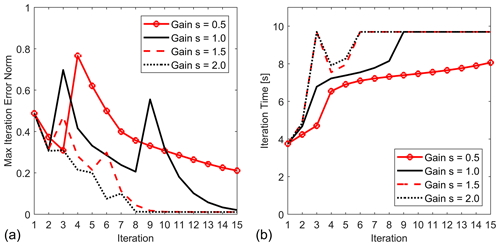Figure 5Convergence comparison of the ILC process for Trajectory A for different values of the multiplier gain s and fine-tuning gain p=0: (a) maximum tracking error and (b) iteration times.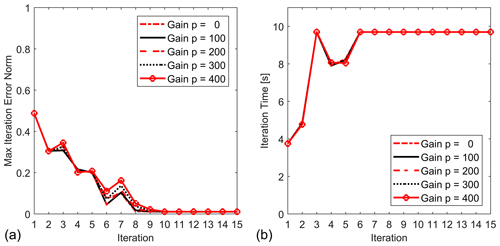Figure 6Convergence comparison of the ILC process for Trajectory A for different values of the fine-tuning gain p and multiplier gain s=2: (a) maximum tracking error and (b) iteration times.

The estimation $\stackrel{\mathrm{^}}{\mathbf{A}}$ of the matrix A which will be used for the initial ILC learning operator will be defined as follows:

$\begin{array}{}\text{(9)}& \begin{array}{rl}& {\stackrel{\mathrm{^}}{a}}_{\mathrm{11}}\left({q}_{\mathrm{2}}\right)=\mathrm{6600}+\mathrm{3000}\mathrm{cos}\left({q}_{\mathrm{2}}\right)\phantom{\rule{0.125em}{0ex}}\mathrm{kg}\phantom{\rule{0.125em}{0ex}}{\mathrm{cm}}^{\mathrm{2}}\\ & {\stackrel{\mathrm{^}}{a}}_{\mathrm{12}}\left({q}_{\mathrm{2}}\right)=\mathrm{1400}+\mathrm{1500}\mathrm{cos}\left({q}_{\mathrm{2}}\right)\phantom{\rule{0.125em}{0ex}}\mathrm{kg}\phantom{\rule{0.125em}{0ex}}{\mathrm{cm}}^{\mathrm{2}}\\ & {\stackrel{\mathrm{^}}{a}}_{\mathrm{21}}\left({q}_{\mathrm{2}}\right)={\stackrel{\mathrm{^}}{a}}_{\mathrm{12}}\left({q}_{\mathrm{2}}\right)\phantom{\rule{0.125em}{0ex}}\mathrm{kg}\phantom{\rule{0.125em}{0ex}}{\mathrm{cm}}^{\mathrm{2}}\\ & {\stackrel{\mathrm{^}}{a}}_{\mathrm{22}}\left({q}_{\mathrm{2}}\right)=\mathrm{1400}\phantom{\rule{0.125em}{0ex}}\mathrm{kg}\phantom{\rule{0.125em}{0ex}}{\mathrm{cm}}^{\mathrm{2}}.\end{array}\end{array}$

When a payload with inertia about its centre of mass Im and mass m is attached, the inertia matrix $\stackrel{\mathrm{‾}}{\mathbf{A}}$ of the simulated robot will be altered in accordance with the payload as follows:

$\begin{array}{}\text{(10)}& \begin{array}{rl}{\stackrel{\mathrm{‾}}{a}}_{\mathrm{11}}\left({q}_{\mathrm{2}}\right)& =\phantom{\rule{0.25em}{0ex}}\mathrm{6596.2}+\mathrm{3011.2}\mathrm{cos}\left({q}_{\mathrm{2}}\right)+{I}_{\mathrm{m}}\\ & +m\left(\mathrm{1109}+\mathrm{1100}\mathrm{cos}\left({q}_{\mathrm{2}}\right)\right)\phantom{\rule{0.125em}{0ex}}\mathrm{kg}\phantom{\rule{0.125em}{0ex}}{\mathrm{cm}}^{\mathrm{2}}\\ {\stackrel{\mathrm{‾}}{a}}_{\mathrm{12}}\left({q}_{\mathrm{2}}\right)& =\phantom{\rule{0.25em}{0ex}}\mathrm{1353.4}+\mathrm{1505.6}\mathrm{cos}\left({q}_{\mathrm{2}}\right)+{I}_{\mathrm{m}}\\ & +m\left(\mathrm{484}+\mathrm{550}\mathrm{cos}\left({q}_{\mathrm{2}}\right)\right)\phantom{\rule{0.125em}{0ex}}\mathrm{kg}\phantom{\rule{0.125em}{0ex}}{\mathrm{cm}}^{\mathrm{2}}\\ {\stackrel{\mathrm{‾}}{a}}_{\mathrm{21}}\left({q}_{\mathrm{2}}\right)& =\phantom{\rule{0.25em}{0ex}}{\stackrel{\mathrm{‾}}{a}}_{\mathrm{12}}\left({q}_{\mathrm{2}}\right)\phantom{\rule{0.125em}{0ex}}\mathrm{kg}\phantom{\rule{0.125em}{0ex}}{\mathrm{cm}}^{\mathrm{2}}\\ {\stackrel{\mathrm{‾}}{a}}_{\mathrm{22}}\left({q}_{\mathrm{2}}\right)& =\phantom{\rule{0.25em}{0ex}}\mathrm{1353.4}+{I}_{\mathrm{m}}+\mathrm{484}m\phantom{\rule{0.125em}{0ex}}\mathrm{kg}\phantom{\rule{0.125em}{0ex}}{\mathrm{cm}}^{\mathrm{2}}.\end{array}\end{array}$

The trajectories used for adaptation (Trajectory A) and for evaluation (Trajectory E) of the optimized learning operator are shown in Fig. 4. They are trajectories with similar characteristics. It is supposed that after the ILC learning operator is adapted for Trajectory A, then the ILC process will have a better performance (faster convergence rate) when the robot must be trained for execution of Trajectory E.

The numerical experiments consider two types of payloads, Payloads A and B, with the following characteristics: Im= 150 kg cm2 and m= 7.5 kg for Payload A and Im= 52.8 kg cm2 and m=2.5 kg for Payload B.

During the initial adaption for Payload A the following values for the multiplier gain s are considered: 0.5, 1.0, 1.5, and 2.0. The fine-tuning gain p is set to 0. The COILC is executed with the desired trajectory set to Trajectory A. The convergence of the ILC process is shown in Fig. 5.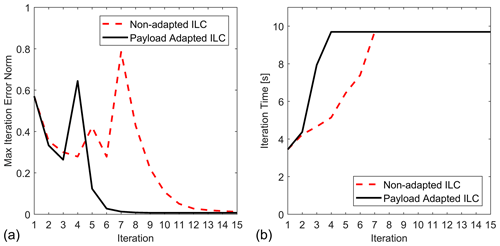Figure 7Convergence comparison of the ILC process for Trajectory E for non-adapted ILC and ILC adapted to the payload: (a) maximum tracking error and (b) iteration times.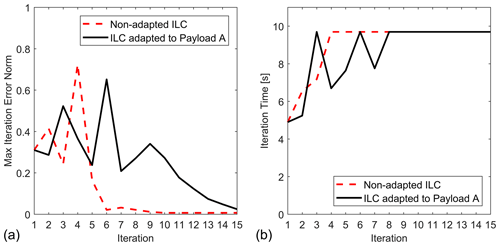Figure 8Convergence comparison of the ILC process for Trajectory E with Payload B for non-adapted ILC and ILC adapted to Payload A: (a) maximum tracking error and (b) iteration times.

Further, the multiplier gain s is set to the best value found of 2.0, and additional adaptation is done by considering the following values for the fine-tuning gain p: 0, 100, 200, 300, and 400. The convergence results are shown in Fig. 6.

Figure 7 shows comparison of the generated ILC process when the desired trajectory is set to Trajectory E. The results show that the total ILC execution time is reduced from 109 to 55 s, which is about a 50 % faster ILC process with the learning operator that is adapted to Payload A with gains s=2 and p=0.

The next experiments are conducted for Payload B. Figure 8 shows the ILC convergence for Trajectory E when non-adapted ILC and ILC adapted to Payload A is used. It can be concluded that with the learning operator adapted to Payload A, the ILC process will need a very high number of iterations for trajectory tracking with Payload B. The ILC process will still be convergent, but the number of iterations will exceed more than 20.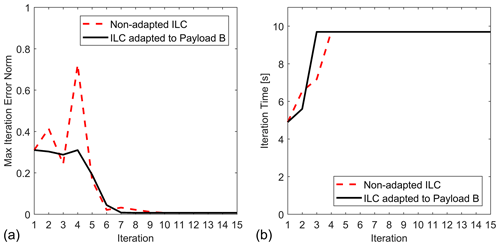Figure 9Convergence comparison of the ILC process for Trajectory E with Payload B for non-adapted ILC and ILC adapted to Payload B: (a) maximum tracking error and (b) iteration times.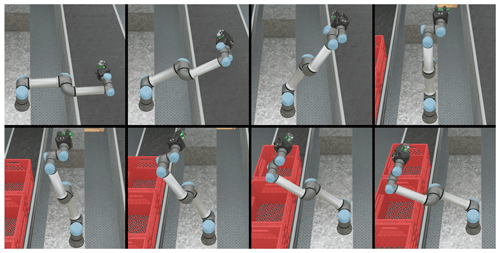Figure 10Positions of the simulated movement of the UR5e robot holding the payload in the gripper.

For the next experiment, a computer simulation is created. For the computer simulation, we consider trajectory movement of the UR5e with a duration of 2 s. All the joints of the robot are fixed, except the second one (the elbow lift joint), which moves from 0.01 rad to 2.60 rad. The robot is in its maximum extended state, which requires the maximum torque when the robot holds the payload in its gripper. For the experiment, two different payloads with a mass of 1 and 7 kg are considered. Some positions from the simulated robot movement are shown in Fig. 10.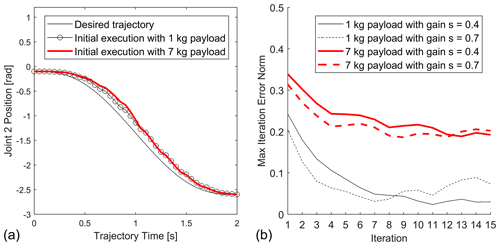Figure 11(a) Desired trajectory and its execution with different payloads. (b) Comparison of the convergence of the ILC process with different payloads and different values of the multiplier gain s.

The desired trajectory of the second link is shown with a thin solid black line in Fig. 11a. The trajectory executions with the two different payloads with the default robot control without applying ILC are shown in Fig. 11a. For the computer simulation, multiple ILC procedures are executed with different values of the multiplier gain. In Fig. 11b the ILC executions are shown for values 0.4 and 0.7 of the multiplier adaptation gain.

These results clearly show that for different payloads, a different multiplier adaptation gain will change the behaviour of the ILC process. For the payload of 1 kg, we can conclude the higher multiplier gain s=0.7 will result in a non-convergent ILC process. For the higher payload of 1 kg, both 0.4 and 0.7 gains will preserve the convergence of the ILC process, but the higher gain will need 9 iterations instead of 13 iterations for the lower to achieve the same value of maximum tracking error. For both payloads, we can use a gain value of 0.4, and the ILC process will minimize the trajectory tracking error. But with the payload adaptation proposed in this research, the overall performance of the ILC can be improved when the payload is also taken into consideration by the robot control. Fewer iterations will be needed for minimizing the trajectory tracking error.

4 Conclusion

Data availability

All the data used in this paper can be obtained from the corresponding author upon request.

Author contributions

KY and LM designed the proposed approach algorithm and experiments. LM carried them out and prepared the results. KY prepared the manuscript with contributions from all co-authors.

Competing interests

The contact author has declared that neither they nor their co-author has any competing interests.

Disclaimer

Publisher's note: Copernicus Publications remains neutral with regard to jurisdictional claims in published maps and institutional affiliations.

Special issue statement

Acknowledgements

The research presented in this paper was supported by the Bulgarian National Science Fund under contract no. KP-06-M47/5-27.11.2020.

Financial support

This research has been supported by the Bulgarian National Science Fund (grant no. KP-06-M47/5-27.11.2020).

Review statement

This paper was edited by Mohamed Amine Laribi and reviewed by two anonymous referees.

References

Arimoto, S., Kawamura, S., and Miyazaki, F.: Iterative learning control for robot systems, in: Proceedings of IECON, Tokyo, Japan, 1984a (in Japanese).

Arimoto, S., Kawamura, S., and Miyazaki, F.: Bettering operation of robots by learning, J. Robotic Syst., 1, 123–140, https://doi.org/10.1002/rob.4620010203, 1984b.

Delchev, K.: Simulation-based design of monotonically convergent iterative learning control for nonlinear systems, Archives of Control Sciences, 22, 371–384, https://doi.org/10.2478/v10170-011-0036-9, 2012.

Eaton, J. W., Bateman, D., Hauberg, S., and Wehbring, R.: GNU Octave version 5.2.0 manual: a high-level interactive language for numerical computations, https://octave.org/doc/octave-5.2.0.pdf (last access: 29 April 2022), 2019.

Heizinger, G., Fenwick, D, Paden, B., and Miyazaki, F.: Robust Learning Control, in: Proc. of 28th Conference on Decision and Control, 13–15 December 1989, Tampa, FL, https://doi.org/10.1109/CDC.1989.70152, 1989.

Hu, J., Li, C., Chen, Z., and Yao, B.: Precision Motion Control of a 6-DoFs Industrial Robot With Accurate Payload Estimation, IEEE-ASME T. Mech., 25, 1821–1829, https://doi.org/10.1109/TMECH.2020.2994231, 2020.

Lee, J., Chang, P. H., and Jin, M.: An Adaptive Gain Dynamics for Time Delay Control Improves Accuracy and Robustness to Significant Payload Changes for Robots, IEEE T. Ind. Electron., 67, 3076–3085, https://doi.org/10.1109/TIE.2019.2912774, 2020a.

Lee, J., P. H. Chang, P. H., Yu, B., and Jin, M.: An Adaptive PID Control for Robot Manipulators Under Substantial Payload Variations, IEEE Access, 8, 162261–162270, https://doi.org/10.1109/ACCESS.2020.3014348, 2020b.

Longman, R. and Huang, Y.: The Phenomenon of Apparent Convergence Followed by Divergence in Learning and Repetitive Control, Intell. Autom. Soft Co., 8, 107–128, https://doi.org/10.1080/10798587.2002.10644210, 2002.

Michel, O.: Cyberbotics Ltd. Webots™: Professional Mobile Robot Simulation, Int. J. Adv. Robot. Syst., 1, 39–42, https://doi.org/10.5772/5618, 2004.

Nemec, B., Simonič, M., Likar, N., and Ude, A.: Enhancing the performance of adaptive iterative learning control with reinforcement learning, in: 2017 IEEE/RSJ International Conference on Intelligent Robots and Systems (IROS), 24–28 September 2017, Vancouver, BC, 2192–2199, https://doi.org/10.1109/IROS.2017.8206038, 2017.

Patan, K., Patan, M., and Kowalów, D.: Neural networks in design of iterative learning control for nonlinear systems, IFAC PapersOnLine, 50, 13402–13407, https://doi.org/10.1016/j.ifacol.2017.08.2277, 2017.

Sekimoto, M., Kawamura, S., Ishitsubo, T, Akizuki, S., and Mizuno, M.: Basis-motion torque composition approach: generation of feedforward inputs for control of multi-joint robots, in: 2009 IEEE/RSJ International Conference on Intelligent Robots and Systems, 10–15 October 2009, St. Louis, MO, USA, 3127–3132, https://doi.org/10.1109/IROS.2009.5354729, 2009.

Shinji, W. and Mita, T.: A Parameter Identification Method of Horizontal Robot Arms, Adv. Robotics, 4, 337–352, https://doi.org/10.1163/156855390X00170, 1990.

Tanimoto, N., Sekimoto, M., Kawamura, S., and Kimura, H.: Generation of feedforward torque by reuse of ILC torque for three-joint robot arm in gravity, in: 2017 56th Annual Conference of the Society of Instrument and Control Engineers of Japan (SICE), 19–22 September 2017, Kanazawa, Japan, 927–931, https://doi.org/10.23919/SICE.2017.8105507, 2017.

Truong, H. V. A., Tran, D. T., To, X. D., Ahn, K. K., and Jin, M.: Adaptive Fuzzy Backstepping Sliding Mode Control for a 3-DOF Hydraulic Manipulator with Nonlinear Disturbance Observer for Large Payload Variation, Appl. Sci., 9, 3290, https://doi.org/10.3390/app9163290, 2019.

Uchiyama, M.: Formulation of high-speed motion pattern of a mechanical arm by trial, Trans. SICE (Soc. Instrum. Contr. Eng.), 14, 706–712, https://doi.org/10.9746/sicetr1965.14.706, 1978.

Wang, Y. C., Chien, C. J., and Lee, D. T.: A fuzzy neural network direct adaptive iterative learning controller for robotic systems, IFAC Proceedings Volumes, 41, 6519–6524, https://doi.org/10.3182/20080706-5-KR-1001.01099, 2008.

Wang, Y., Chien, C., and Chi, R.: A fuzzy neural network based adaptive terminal iterative learning control for nonaffine nonlinear discrete-time systems, in: Proceedings of the 2014 International Conference on Fuzzy Theory and Its Applications (iFUZZY2014), 26–28 November 2014, Kaohsiung, Taiwan, https://doi.org/10.1109/iFUZZY.2014.7091252, 163–167, 2014.

Wei-Liang, K., Cheng, M., and Lin, H.: Reinforcement Q-Learning and ILC with Self-Tuning Learning Rate for Contour Following Accuracy Improvement of Biaxial Motion Stage, AD Publications, Rajasthan, India, http://ijoer.com/Paper-March-2017/IJOER-MAR-2017-8.pdf (last access: 29 April 2022), 2017.

Xu, P., and Xu, Q.: Neural network-based AILC for nonlinear discrete-time system with iteration varying initial error and reference trajectory, in: Proceedings of the 2018 5th International Conference on Information Science and Control Engineering (ICISCE), 20–22 July 2018, Zhengzhou, China, https://doi.org/10.1109/ICISCE.2018.00179, 854–858, 2018.

Yovchev, K. and Miteva, L.: Preliminary Study of Self-adaptive Constrained Output Iterative Learning Control for Robotic Manipulators, in: Advances in Service and Industrial Robotics. RAAD 2021. Mechanisms and Machine Science, edited by: Zeghloul, S., Laribi, M. A., and Sandoval, J., Springer, Cham, https://doi.org/10.1007/978-3-030-75259-0_30, 2021.

Yovchev, K., Delchev, K., and Krastev, E.: Constrained Output Iterative Learning Control, Archives of Control Sciences, 30, 157–176, https://doi.org/10.24425/acs.2020.132590, 2020a.

Zhang, Y., Chu, B., and Shu, Z.: A Preliminary Study on the Relationship Between Iterative Learning Control and Reinforcement Learning, IFAC PapersOnLine, 52, 314–319, https://doi.org/10.1016/j.ifacol.2019.12.669, 2019.